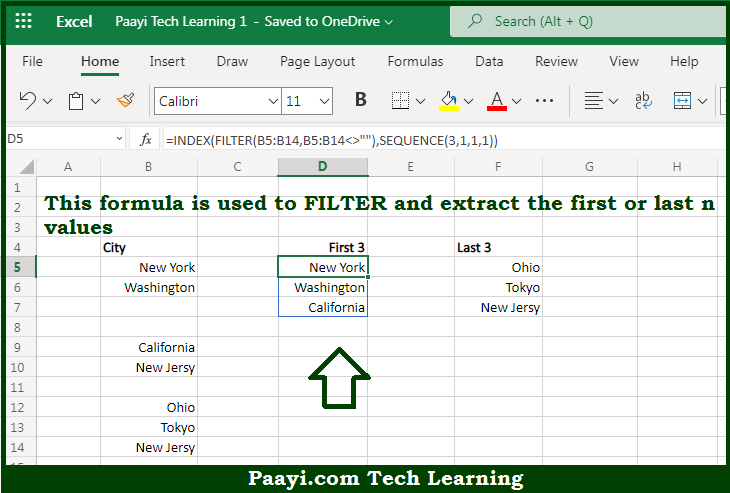# Learn How to Filter On First or Last n Values in Microsoft Excel

Written by | 0 Comments | 325 Views

In this article, you will learn how to evaluate things in Dynamic Arrays with formulas in Microsoft Excel using a single/combination(s) of functions. You will also know How to Filter On First or Last n Values and see the generic formula.

Learn How to Filter On First or Last n Values in Microsoft Excel

The main purpose of this formula is to FILTER and extract the first or last n values that mean the first 3 values, or first 7 values, and so on. Here we will learn how to filter first or last n values in the given data range in the workbook in Microsoft Excel. That implies, with the help of a formula based on the FILTER, INDEX, and SEQUENCE function you can able to FILTER and extract the first or last n values that mean the first 3 values, or first 7 values, and so on. So, with the help of this formula, you can able to filter first or last n values in the given data range in the workbook in Microsoft Excel.

General Formula to Filter On First or Last n Values

=INDEX(FILTER(data,logic),SORT(SEQUENCE(n,1,SUM(--(logic)),-1)),SEQUENCE(1,COLUMNS(data)))

The Explanation for the Filter On First or Last n ValuesSo we know that with the help of the given formula above you can able to FILTER and extract the first or last n values that mean the first 3 values, or first 7 values, and so on. Here we will learn how to filter first or last n values in the given data range in the workbook in Microsoft Excel. As we know that the FILTER function is constructed to filter entries based on a logical test, moreover the INDEX function is used to extract the last 3 entries from the filtered list. Working from the inside out, we use the SEQUENCE function to construct a row number value for the INDEX function. So, with the help of this formula, you can able to FILTER and extract the first or last n values that mean the first 3 values, or first 7 values, and so on. Here we will learn how to filter first or last n values in the given data range in the workbook in Microsoft Excel.